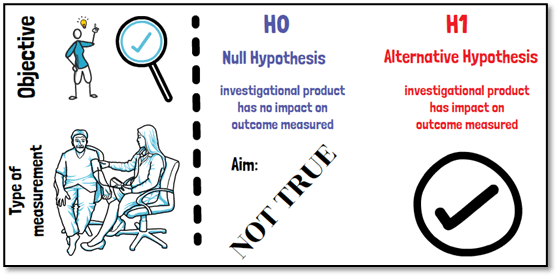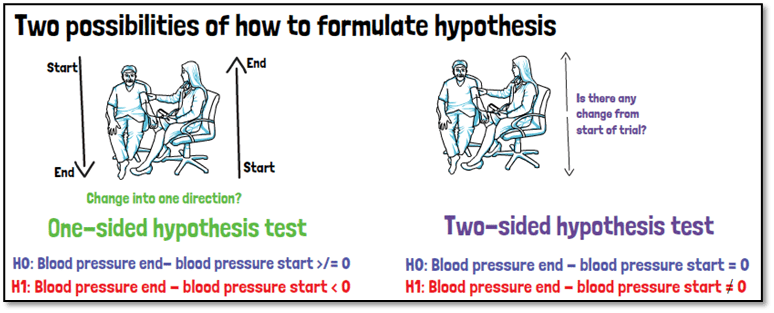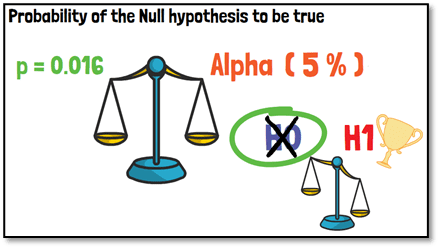# What is the null and alternative hypothesis?When conducting a clinical trial one of the most important aspects is to define the objective of a study and to decide on what the decision in favor of or against the investigational product will be based. It is here where the definition of hypotheses comes into play. Once it is decided what the objective of a clinical trial should be, and how it will be measured, this is when the study hypotheses must be defined.

Hypotheses always come in pairs, called the null hypothesis and the alternative hypothesis. The null hypothesis describes the non-favorable scenario, where the investigational product would have no impact on the outcome measured. It is that scenario opposing the null hypothesis that you would like to support.

Let us take an example of a medication that is supposed to reduce your blood pressure: There are two possibilities of how to formulate your hypotheses, and they depend on your objective. Do you want to prove that the blood pressure is changing into one direction, meaning that you expect it to increase or decreases with time? Or do you not have any expectations and just want to see if there is a change in comparison to the start of the trial? The first approach is called a one-sided hypothesis test. The second approach is called a two-sided hypothesis test.The null hypothesis in both cases would state that there will be no change (two-sided test), in case of the one-sided test additionally a possible positive change in blood pressure between the beginning and end of the trial. An example for a one-sided alternative hypothesis, which we aim to find evidence for, is that the blood pressure decreases over the period of the trial, so that the difference between the two time points is smaller than zero. Whereas the alternative hypothesis for a two-sided test would be to prove that there is a difference between the blood pressures measured at the start and at the end of the trial in either direction.

The result of our statistical test then gives us the probability of the null hypothesis being true in our setting. This probability is then compared to a threshold, called alpha, to see if we can reject the null hypothesis in favor of the alternative hypothesis. Very often alpha is set to be 5% for two-sided hypotheses. Having rejected the null hypothesis based on our probability, does not mean that the alternative hypothesis is true. It just means that it is more likely to be true than the null hypothesis you tested. We say that the null hypothesis can be rejected in favor of the alternative hypothesis.If you are interested in a visual presentation of this topic, please feel free to follow us on our GCP Mindset YouTube channel! If you would like to know more about how we could implement statistics in your clinical trial, send us a mail at statistics@gcp-service.com.